# Lions Weekly: September 8, 2021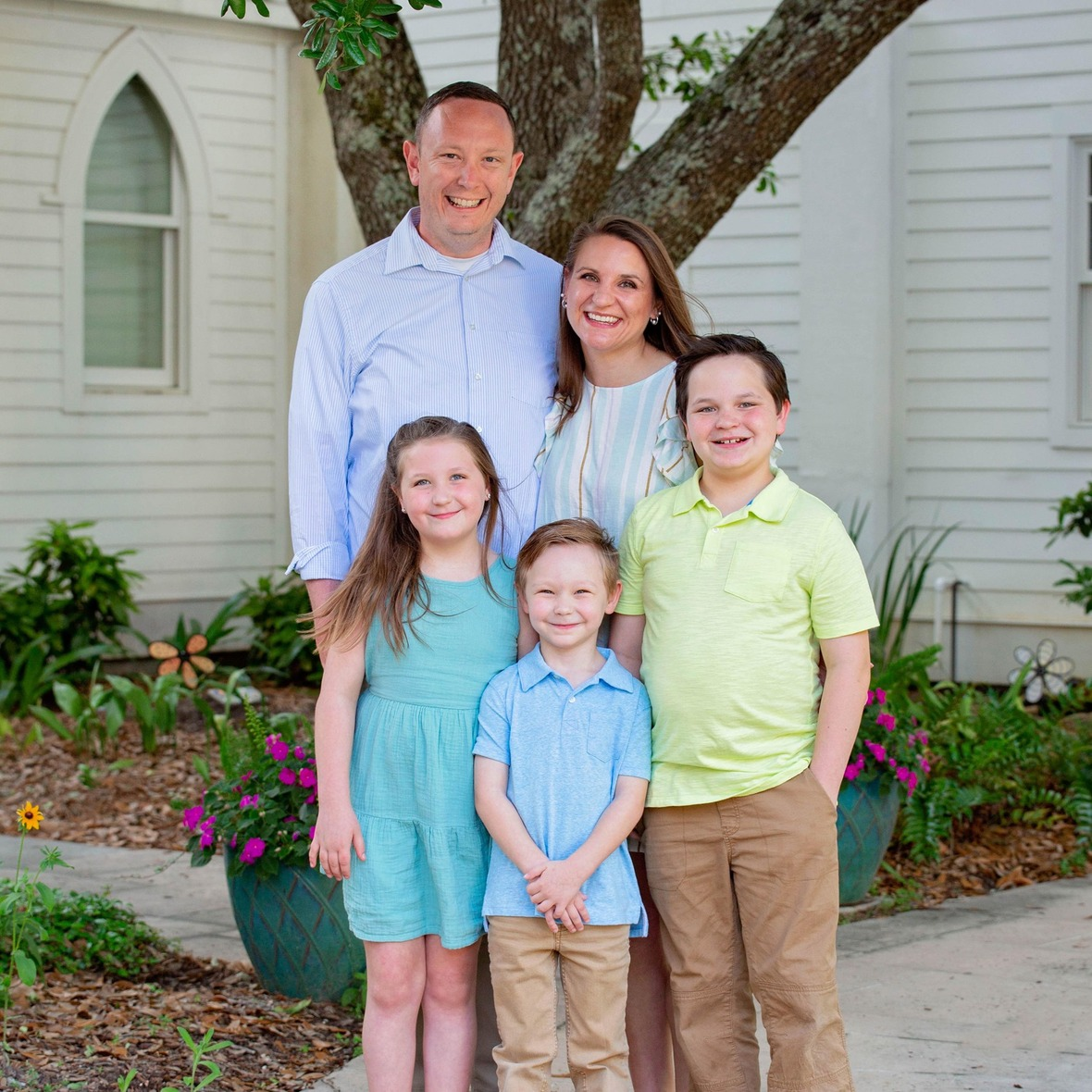# A Note From Mr. Sandefur

Lion family,

As we prepare to celebrate Homecoming this Friday evening, I want to encourage all that are able to, join us for the festivities. Homecoming is a time where we can look back on the past and celebrate the present. With over 140 years of legacy, TKA’s Homecoming is an even more special event as this place is special to many people from different generations. I personally look forward to meeting alumni, family members, and those of you who I have not had the pleasure of meeting during one of our homecoming festivities.

We will host the Homecoming Parade and Pep Rally beginning at 1:30 on Friday afternoon, and all community members, alumni, etc. are invited to participate. We will begin our Homecoming festivities at 7:00 p.m., followed by our game against Grace Christian at 7:30. Friday promises to be full of fun and excitement, so please plan on joining us if you are able. Due to the events being held outside, masks are not required. However, they continue to be encouraged for large gatherings within close proximity.

I hope to see you soon, and Go Lions!

In Christ Alone,

Jeremy Sandefur

President

 table div table+table+table+table div table{width:100%;padding:0}table div table+table+table+table div table img{width:96.23%;padding:0;float:none}table div table+table+table+table div table td{width:100%;padding:0 1.88% 18px}/* styles */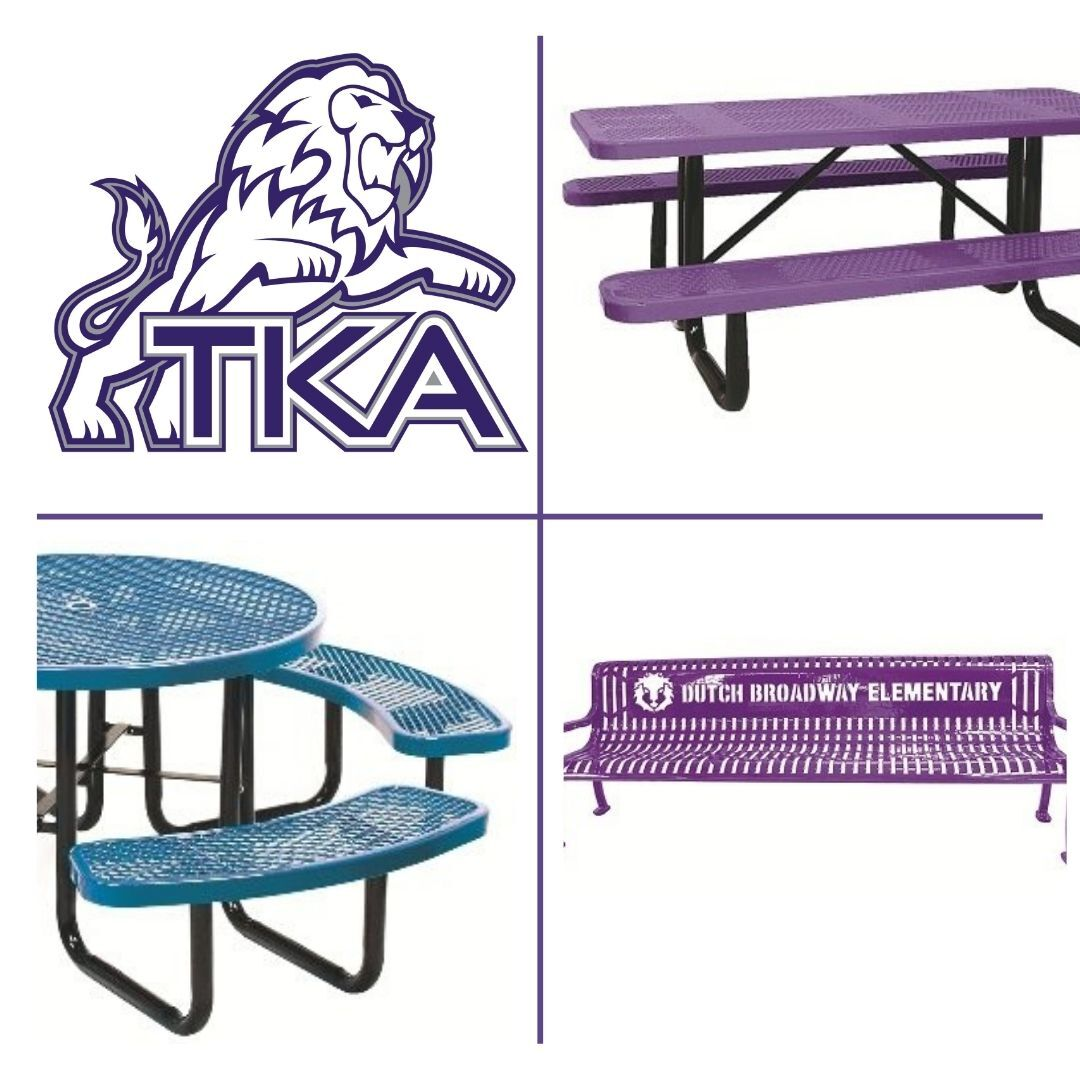# Dr. Carroll Reece Reagan Pavilion

The Dr. Carroll Reece Reagan Pavilion is nearing completion (aiming for the next few weeks), and we are in need of a few donations to furnish the space for our students. Upon completion, the Reagan Pavilion will be a greatly used outdoor spot on campus for covered meals, class meetings, fellowships, and more. If you, or your company, are interested in meeting one of these needs for our students you may either use the below link (list Pavilion table in the note) or contact the school office at (865) 573-8321. The following choices have been made based on durability and function. These will greatly add to this exciting spot on campus! All sponsors are greatly appreciated and will be listed within the Pavilion.

10ft purple tables (6 tables, 1 already sponsored leaving a need for 5)
\$1,300

46 inch round purple tables
\$850

Personalized Benches

6ft benches (2 benches) \$1,200

 table div table+table+table+table+table+table div table{width:100%;padding:0}table div table+table+table+table+table+table div table img{width:96.23%;padding:0;float:none}table div table+table+table+table+table+table div table td{width:100%;padding:0 1.88% 18px}/* styles */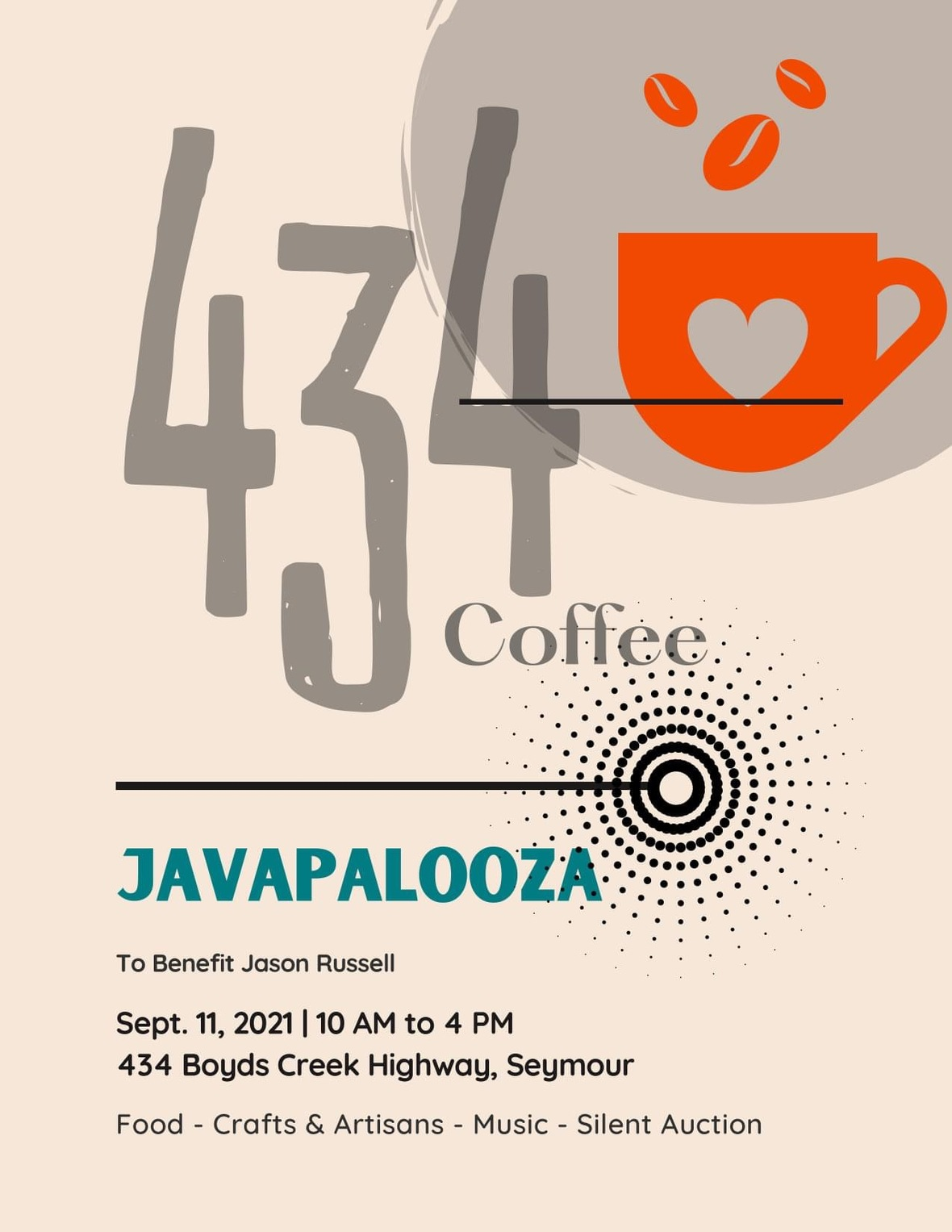# Love Where We Live

The next opportunity we have to partner with our community is a benefit for the the 434 Coffee family. The owners of 434 Coffee are Jason and Jynnifer Russell. Jason was diagnosed with COVID-19 and had been in the ICU. A Javapalooza has been planned for this Saturday, September 11 from 10AM to 4PM at 434 Boyds Creek Highway. The Javaplooza will include food, crafts & artisans, music, and a silent auction. We would love to see as many Lion families at this event as possible as we meet the needs of those around us!

Thank you for helping us Love Where We Live!

 table div table+table+table+table+table+table+table+table div table{width:100%;padding:0}table div table+table+table+table+table+table+table+table div table img{width:96.23%;padding:0;float:none}table div table+table+table+table+table+table+table+table div table td{width:100%;padding:0 1.88% 18px}/* styles */# TKA Alumni

The King's Academy would like to invite our Alumni to experience some of the Homecoming events happening on campus this Friday, September 10!
Come join us for the parade at 1:30 p.m. with a pep rally to follow. Continue the fun by attending our tailgating event at 5:30 p.m. before the game! If you can't attend these events, we would love to have as many alumni as possible cheering on our Lions in the stands as they take on Grace Christian!

 table div table+table+table+table+table+table+table+table+table+table div table{width:100%;padding:0}table div table+table+table+table+table+table+table+table+table+table div table img{width:96.23%;padding:0;float:none}table div table+table+table+table+table+table+table+table+table+table div table td{width:100%;padding:0 1.88% 18px}/* styles */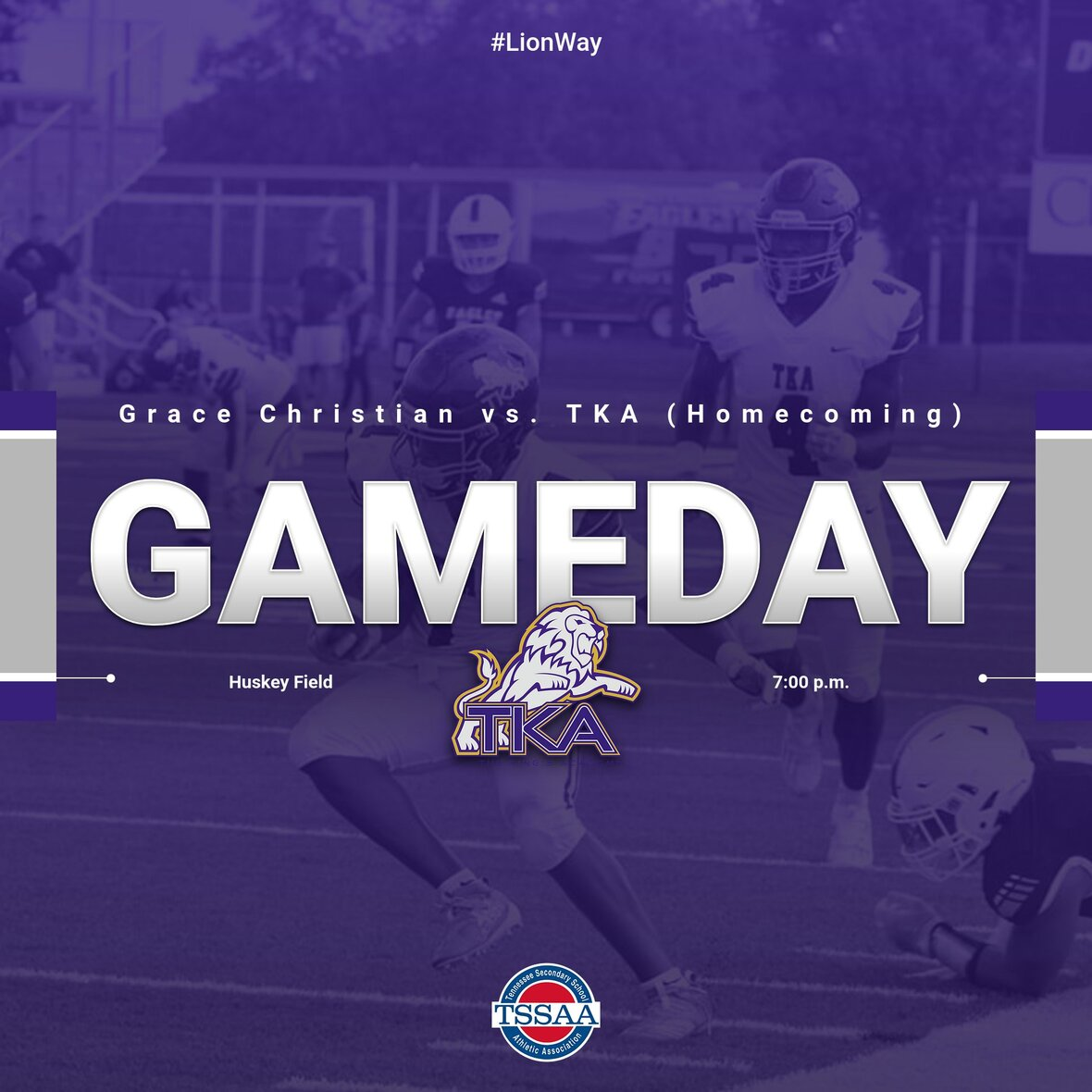# Varsity Football

Our homecoming game is this Friday night! Pre-game activities begin at 5:30 p.m. with tailgating followed by the Homecoming Court Presentation and Coronation Ceremony at 7:00 p.m. Kickoff will take place at 7:30 p.m. Parking is \$5 and tickets are \$8 per person. We look forward to seeing you there as the Lions take on Grace Christian!Face painting spirit dots will be available at the football game Friday night! Support the MS Lady Lions Basketball team from 5:30-kickoff by stopping by and getting your face paint spirit dots!

 /* styles */
 table div table+table+table+table+table+table+table+table+table+table+table+table+table+table div table{width:100%;padding:0}table div table+table+table+table+table+table+table+table+table+table+table+table+table+table div table img{width:96.23%;padding:0;float:none}table div table+table+table+table+table+table+table+table+table+table+table+table+table+table div table td{width:100%;padding:0 1.88% 18px}/* styles */# Homecoming Week: September 7-10

This year's theme is Welcome to The Jungle! Each day students will be allowed to dress up according to the day's theme.

## Antique cars for the Homecoming Parade:

TKA’s Homecoming Parade is always a highlight of the week’s festivities, and we welcome classic or antique cars and/or trucks in the parade. If you have such a vehicle, and you would be willing to drive it in the parade at 1:30 p.m. on Friday, September 10 please contact Mr. Ayers via email at jayers@thekingsacademy.net.

## Full campus activities:

▪ 1:30 p.m. - Homecoming Parade
▪ 2:00 p.m. - Homecoming Pep Rally
▪ 3:00 p.m. - 6:00 p.m. - Book fair open for shopping
▪ 5:30 p.m. - Pre-game tailgating
▪ 7:00 p.m. - Homecoming Court Presentation & Coronation Ceremony
▪ 7:30 p.m. - Football Game v. Grace Christian Academy of Franklin
 ▪ 1:30 p.m. - Homecoming Parade
 ▪ 2:00 p.m. - Homecoming Pep Rally
 ▪ 3:00 p.m. - 6:00 p.m. - Book fair open for shopping
 ▪ 5:30 p.m. - Pre-game tailgating
 ▪ 7:00 p.m. - Homecoming Court Presentation & Coronation Ceremony
 ▪ 7:30 p.m. - Football Game v. Grace Christian Academy of Franklin

## Dress Out Day expectations are as follows:

▪ Dress code length (shorts/skirts, etc.) are expected
▪ Dress code footwear is expected
▪ Jeans are allowed (no rips, tears, etc.)
▪ No inappropriate, vulgar, or any print that goes against the mission and vision of TKA is allowed
▪ Hats are allowed but are to be removed in the classroom if asked to allow all students visual access to presented materials, etc.
 ▪ Dress code length (shorts/skirts, etc.) are expected
 ▪ Dress code footwear is expected
 ▪ Jeans are allowed (no rips, tears, etc.)
 ▪ No inappropriate, vulgar, or any print that goes against the mission and vision of TKA is allowed
 ▪ Hats are allowed but are to be removed in the classroom if asked to allow all students visual access to presented materials, etc.

# Tuesday, September 7: Colors of the Jungle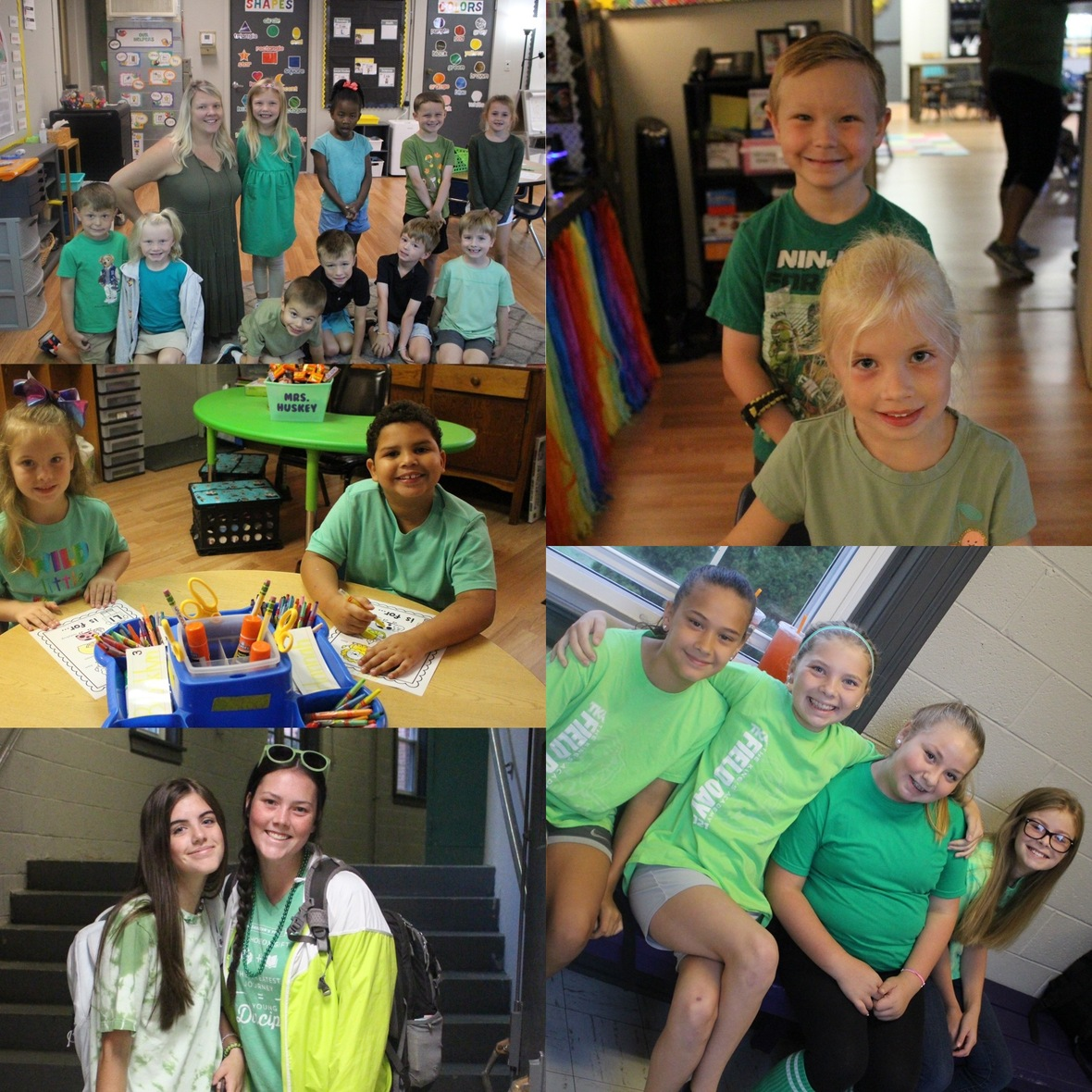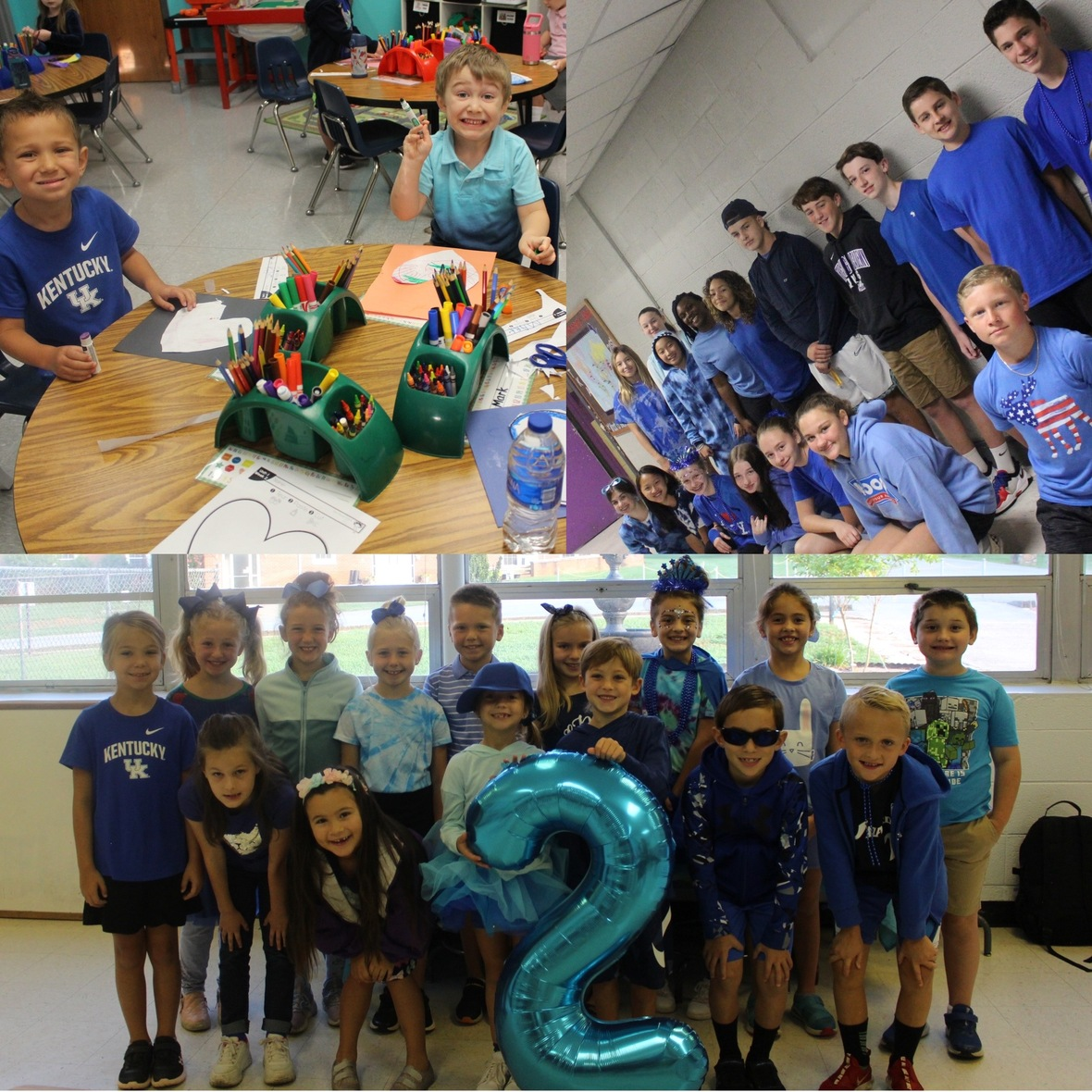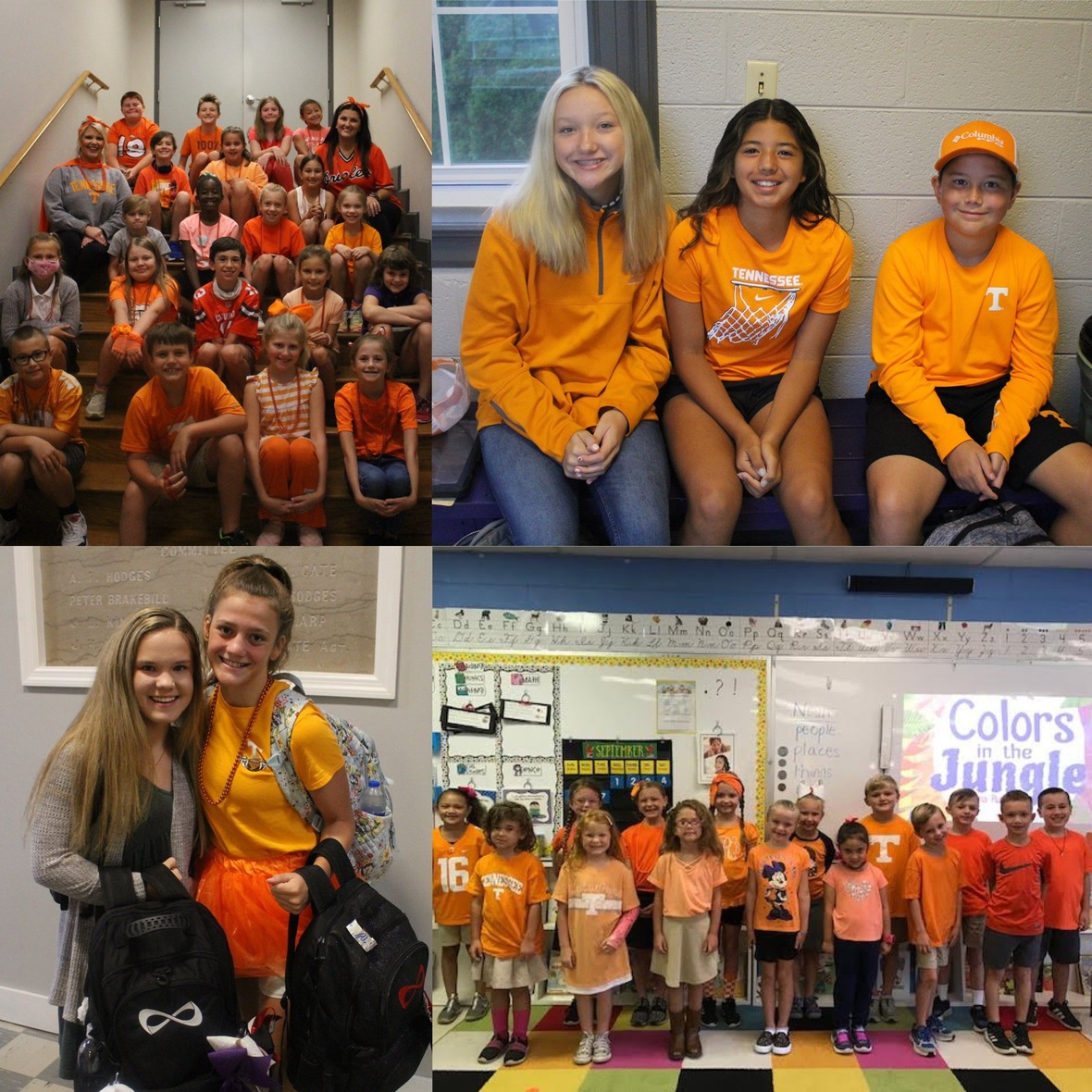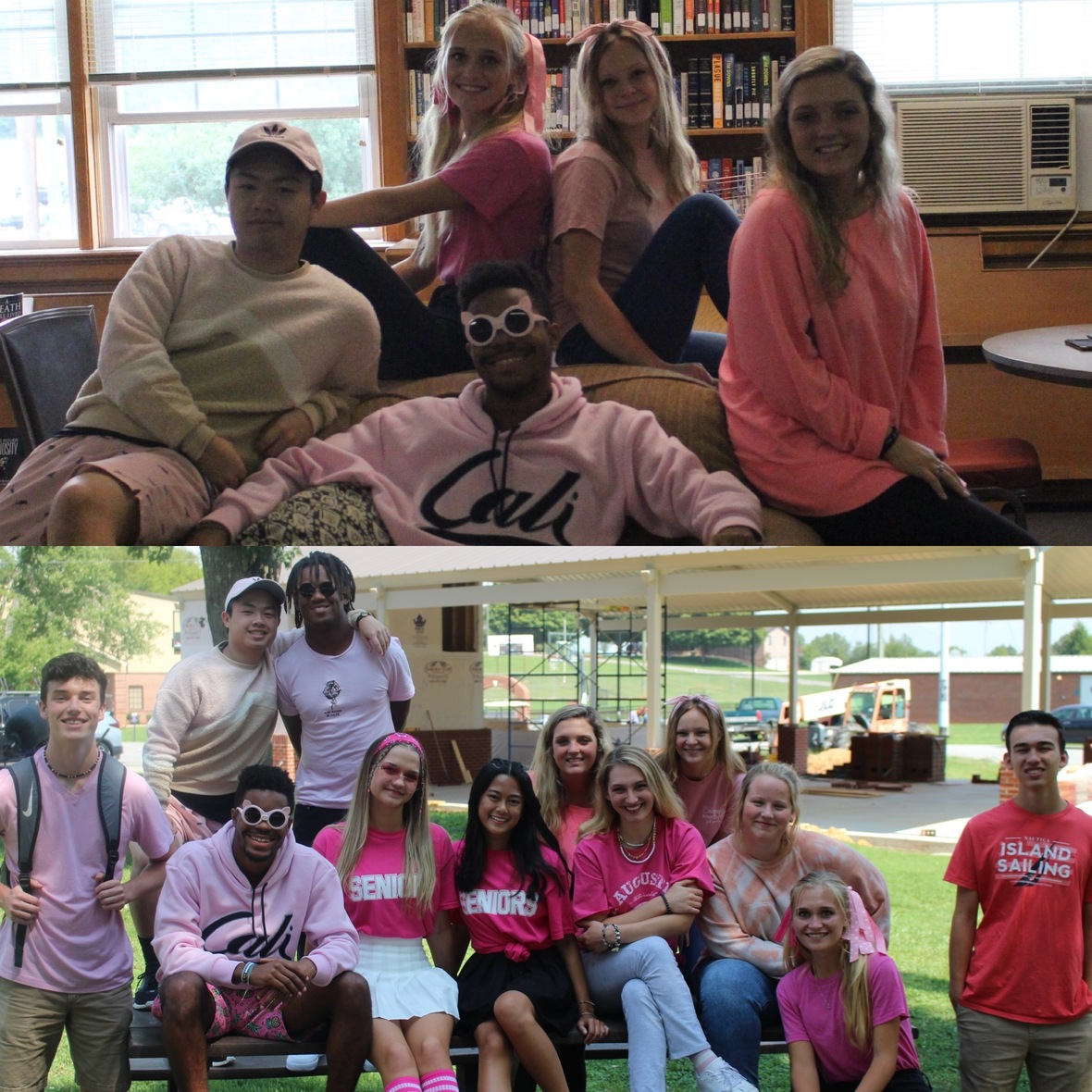# Wednesday, September 8: Movies of the Jungle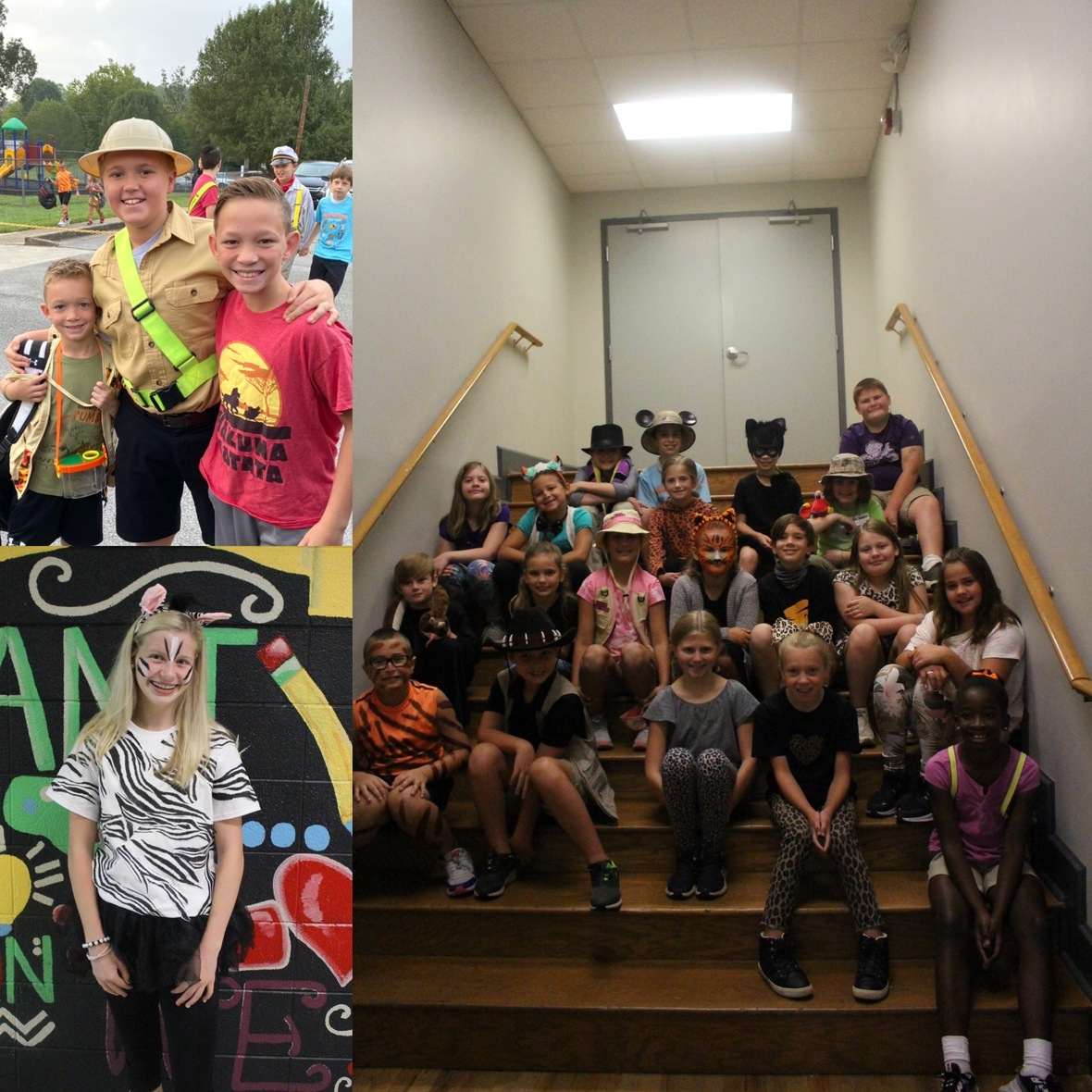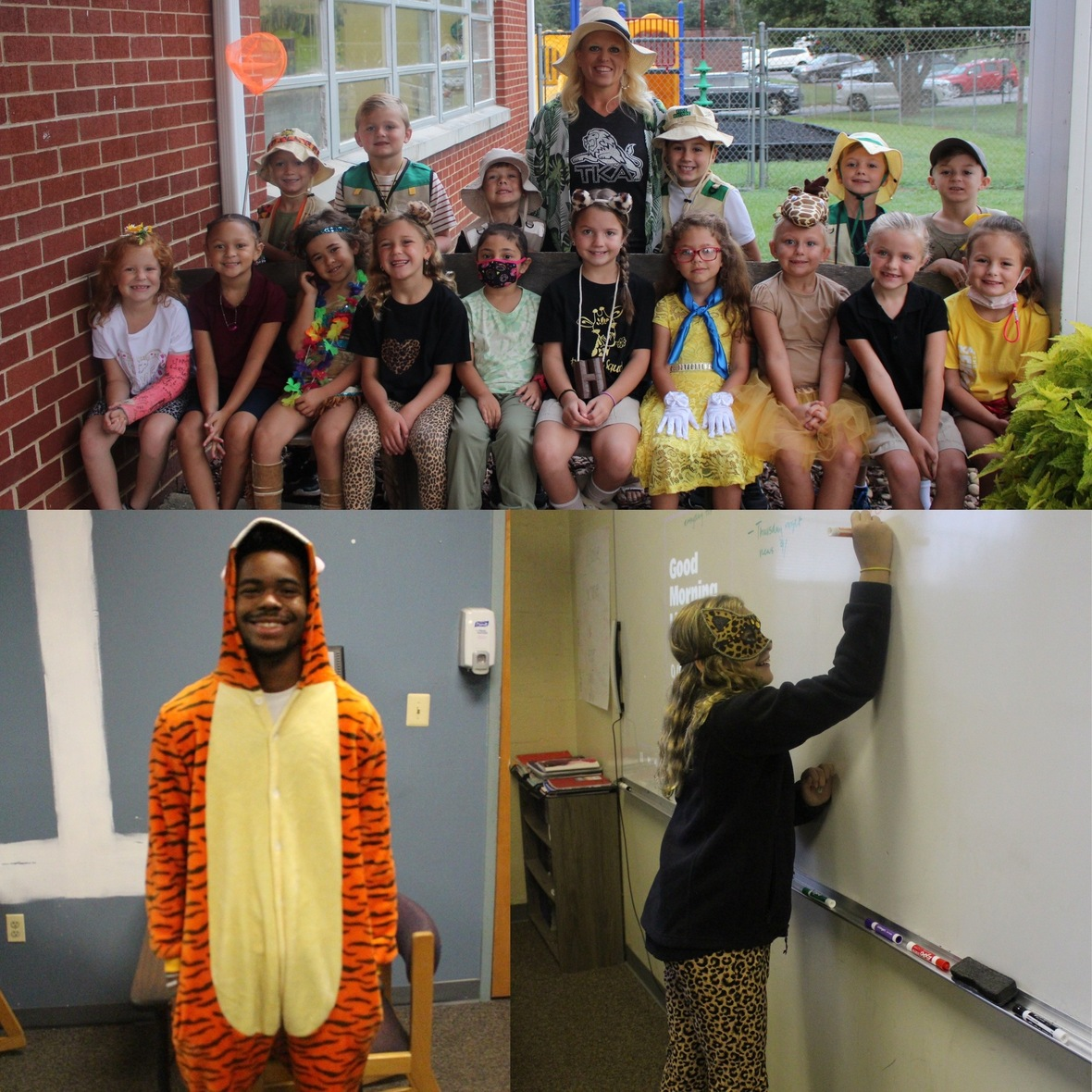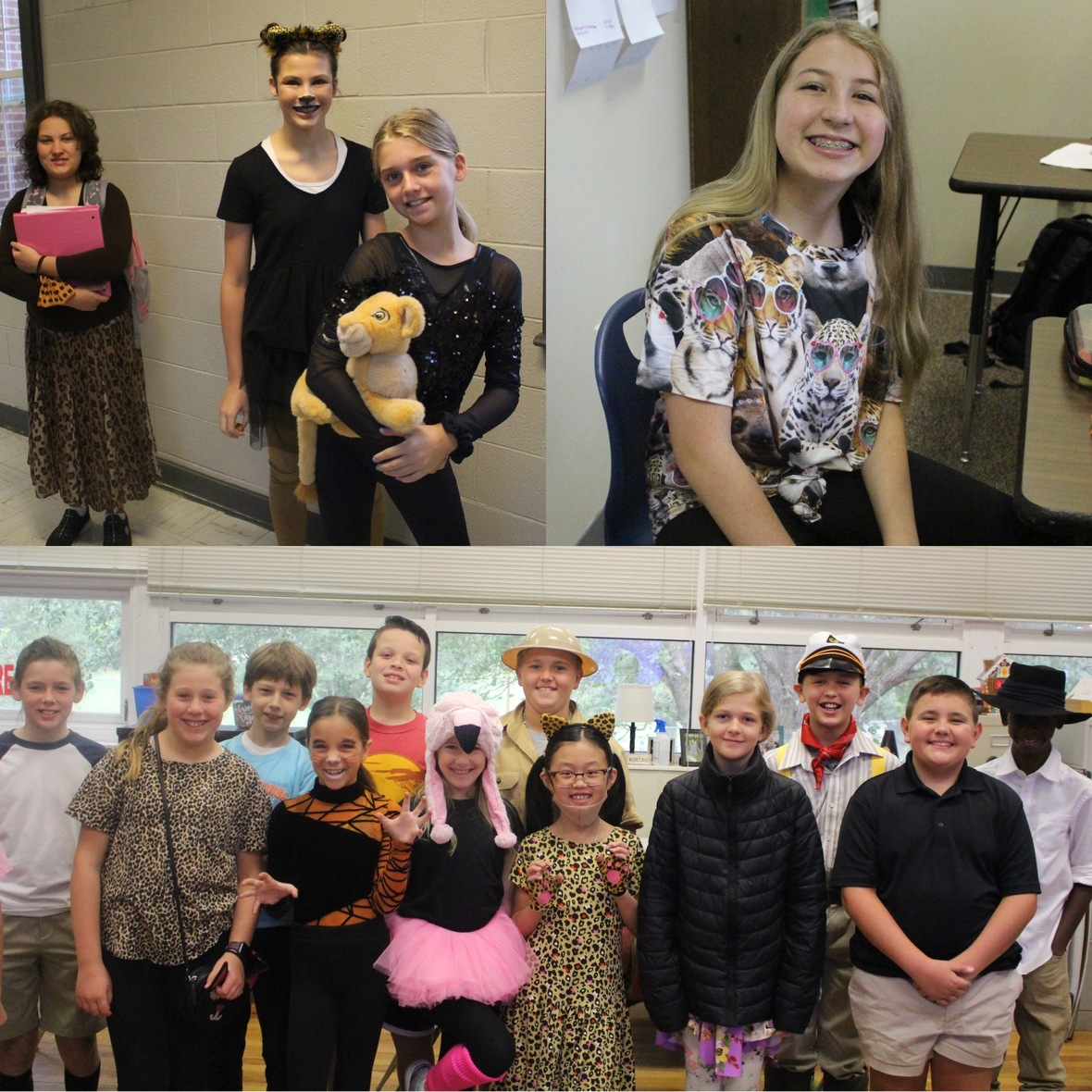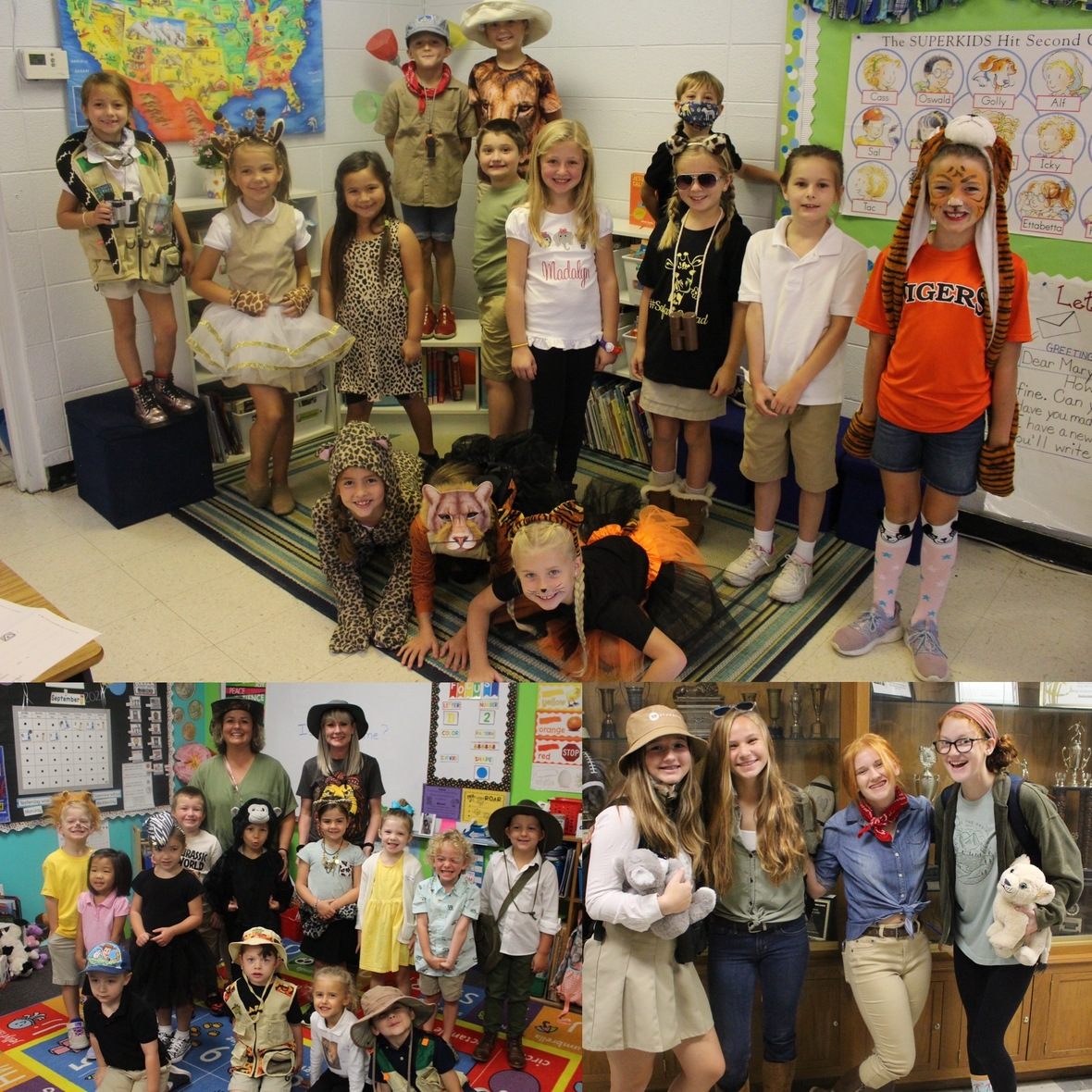# Thursday, September 9:The Lion Sleeps Tonight# Friday, September 10: Purple and Black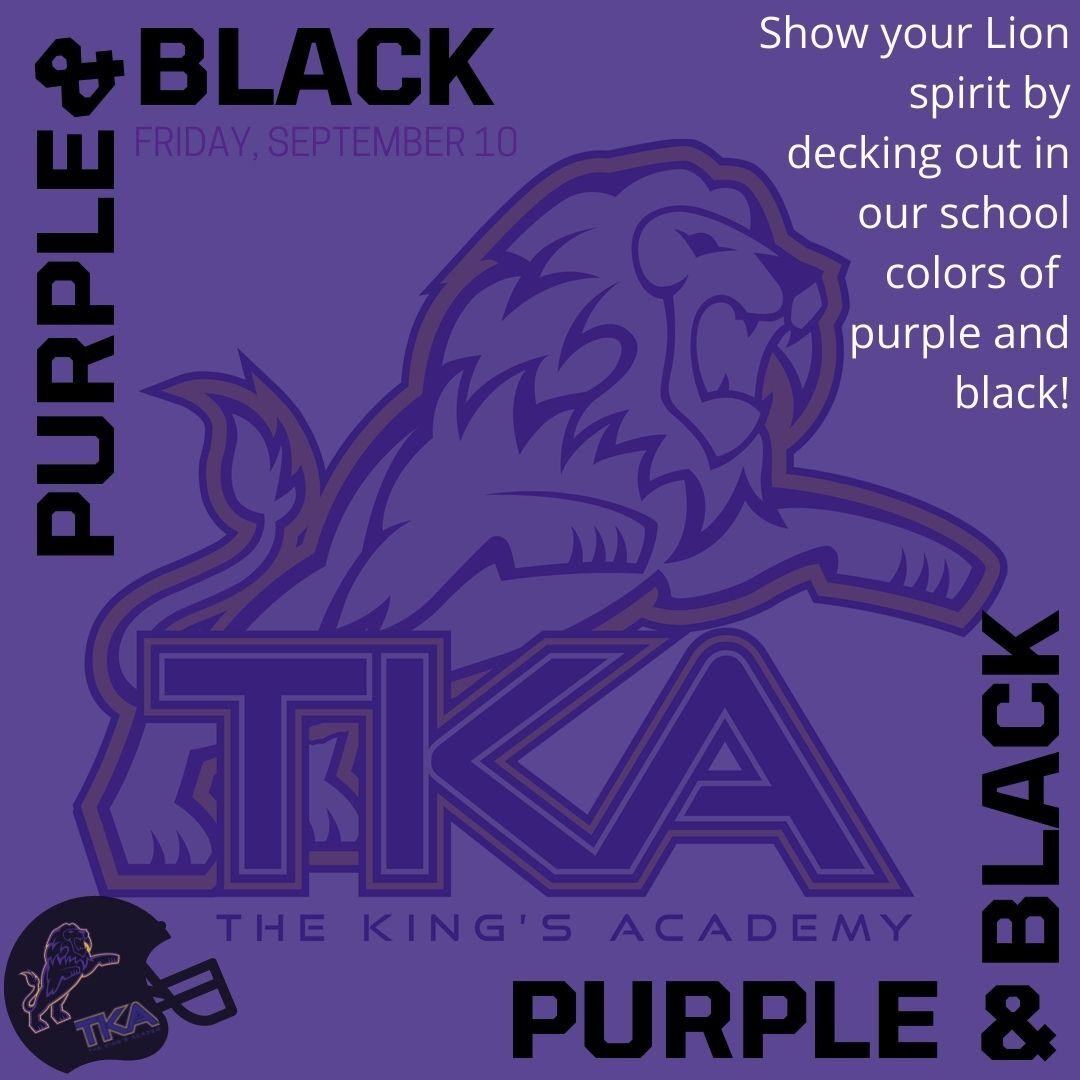table div table+table+table+table+table+table+table+table+table+table+table+table+table+table+table+table+table+table+table+table+table+table+table+table+table+table+table+table+table+table+table div table{width:100%;padding:0}table div table+table+table+table+table+table+table+table+table+table+table+table+table+table+table+table+table+table+table+table+table+table+table+table+table+table+table+table+table+table+table div table img{width:96.23%;padding:0;float:none}table div table+table+table+table+table+table+table+table+table+table+table+table+table+table+table+table+table+table+table+table+table+table+table+table+table+table+table+table+table+table+table div table td{width:100%;padding:0 1.88% 18px}/* styles */# Book Fair

TKA Book Fair begins this Friday, September 10! It will be open after the pep rally from 3:00 PM to 6:00 PM. It will be open the following week, September 13-17. The hours are listed below and more information can be found by clicking here.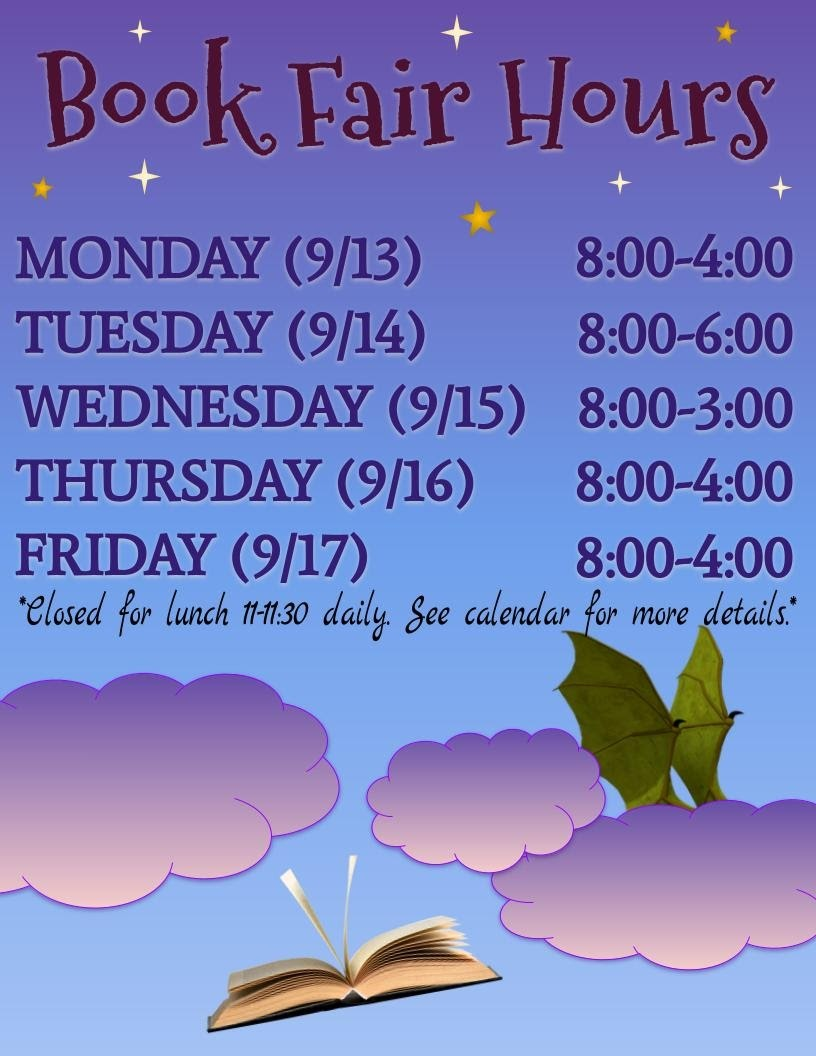table div table+table+table+table+table+table+table+table+table+table+table+table+table+table+table+table+table+table+table+table+table+table+table+table+table+table+table+table+table+table+table+table+table+table div table{width:100%;padding:0}table div table+table+table+table+table+table+table+table+table+table+table+table+table+table+table+table+table+table+table+table+table+table+table+table+table+table+table+table+table+table+table+table+table+table div table img{width:96.23%;padding:0;float:none}table div table+table+table+table+table+table+table+table+table+table+table+table+table+table+table+table+table+table+table+table+table+table+table+table+table+table+table+table+table+table+table+table+table+table div table td{width:100%;padding:0 1.88% 18px}/* styles */# Econ Week: September 13th-17th

Our high school economics class students will be hosting "Econ Week" September 13th - 17th. This activity is a "free enterprise" experience for the students in basic business lessons (budget, competition, profit/loss, etc.). Students will set up "stores" inside and on the walkway entering the cafeteria to sell a variety of goods during lunch, (some groups may choose to sell before/after school. Past stores have sold baked goods, pizza, lunch menu items, doughnuts, etc. Elementary and middle school students will be offered only pre-packaged items along with canned/bottled drinks. Prices generally range from \$0.50 - \$3.00. Students are always eager to buy these items, so you may want to consider reminding them to bring some extra cash during the week. Students keep half of the profits with the other half benefitting approved charities.

 table div table+table+table+table+table+table+table+table+table+table+table+table+table+table+table+table+table+table+table+table+table+table+table+table+table+table+table+table+table+table+table+table+table+table+table+table div table{width:100%;padding:0}table div table+table+table+table+table+table+table+table+table+table+table+table+table+table+table+table+table+table+table+table+table+table+table+table+table+table+table+table+table+table+table+table+table+table+table+table div table img{width:96.23%;padding:0;float:none}table div table+table+table+table+table+table+table+table+table+table+table+table+table+table+table+table+table+table+table+table+table+table+table+table+table+table+table+table+table+table+table+table+table+table+table+table div table td{width:100%;padding:0 1.88% 18px}/* styles */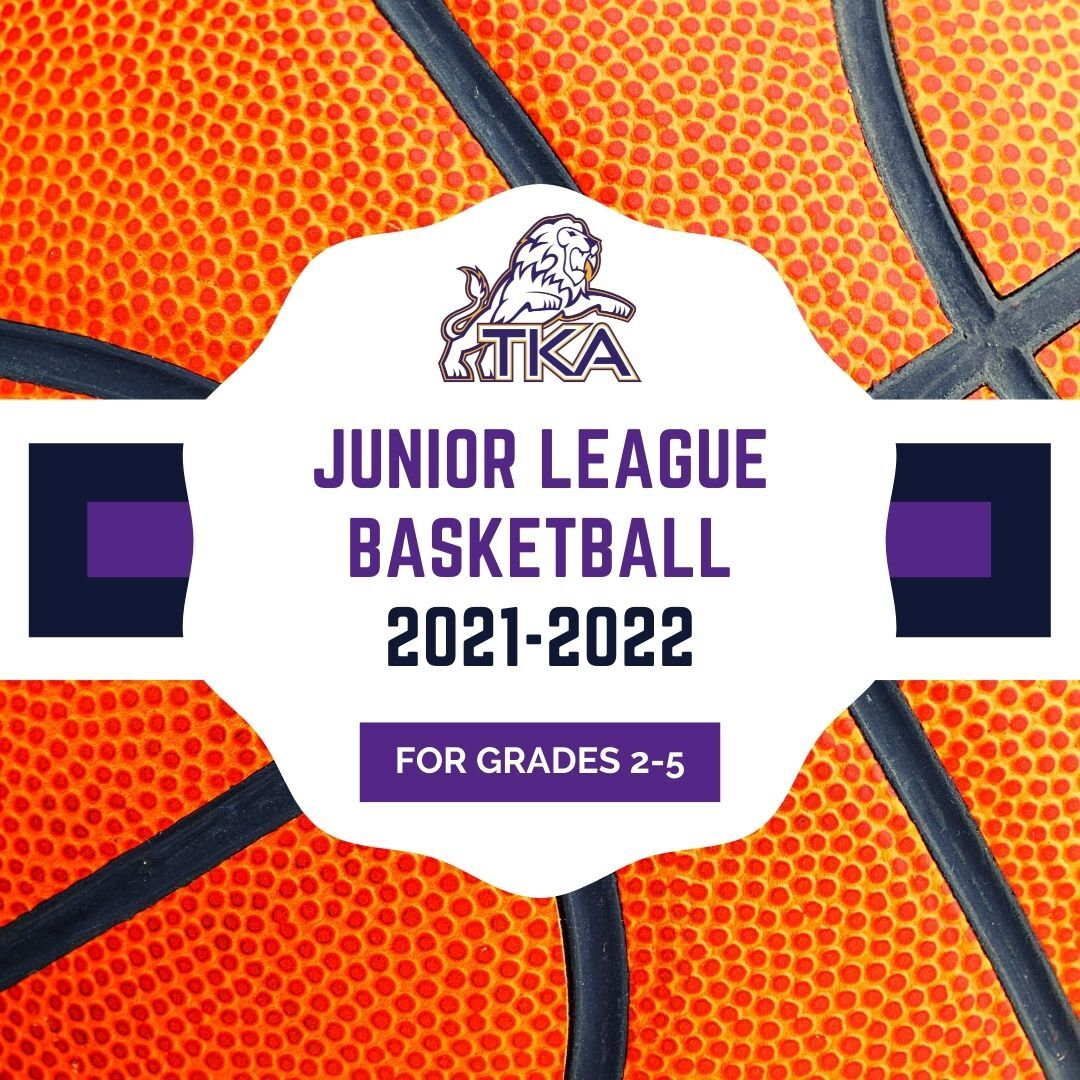Is your child interested in playing basketball for TKA's Jr. League teams this year? Please complete this brief 'TKA Jr. League Basketball Interest Form' that will assist us in determining interest and developing preliminary rosters. Once completed our Jr. League leadership team will evaluate the responses and reach out to you with additional information.

Jr. League is a competitive elementary basketball league which typically consists of 1-2 practices per week. Historically there are 10 regular season games which take place on Saturdays in gyms throughout Sevier County (TKA included). The season typically begins in early November and concludes around mid-February.

We look forward to hearing from you!

Marshall Stanger
TKA Junior League Director
tkahoops@gmail.com
865.566.7928

 table div table+table+table+table+table+table+table+table+table+table+table+table+table+table+table+table+table+table+table+table+table+table+table+table+table+table+table+table+table+table+table+table+table+table+table+table+table+table div table{width:100%;padding:0}table div table+table+table+table+table+table+table+table+table+table+table+table+table+table+table+table+table+table+table+table+table+table+table+table+table+table+table+table+table+table+table+table+table+table+table+table+table+table div table img{width:96.23%;padding:0;float:none}table div table+table+table+table+table+table+table+table+table+table+table+table+table+table+table+table+table+table+table+table+table+table+table+table+table+table+table+table+table+table+table+table+table+table+table+table+table+table div table td{width:100%;padding:0 1.88% 18px}/* styles */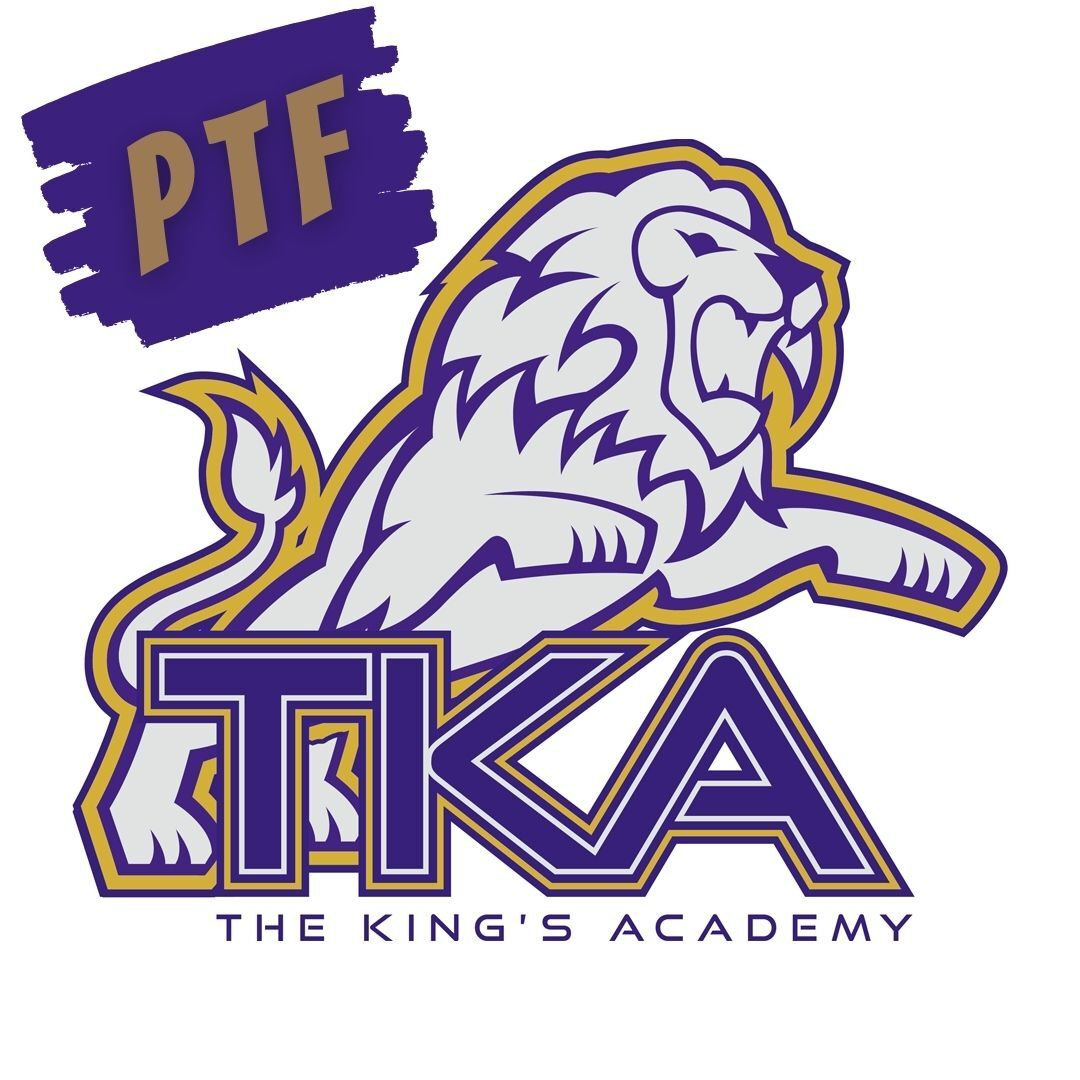# PTF

The next PTF meeting will take place on Thursday, September 16th from 3:15PM-4:05PM in Brandes. Childcare will be provided through out aftercare program free of charge until 4:15PM for the meeting.

If you haven't joined PTF, no worries, come anyway! Membership forms will be available at the meeting. The fee to join is \$25 per family. We look forward to having you as part of our PTF this year!

 table.module-39{width:99.81%;padding:0}table div table+table+table+table+table+table+table+table+table+table+table+table+table+table+table+table+table+table+table+table+table+table+table+table+table+table+table+table+table+table+table+table+table+table+table+table+table+table+table+table div table{width:99.81%;float:none;margin-left:auto;margin-right:auto;padding:0}table div table+table+table+table+table+table+table+table+table+table+table+table+table+table+table+table+table+table+table+table+table+table+table+table+table+table+table+table+table+table+table+table+table+table+table+table+table+table+table+table div table a{border:0 none;text-decoration:none}table div table+table+table+table+table+table+table+table+table+table+table+table+table+table+table+table+table+table+table+table+table+table+table+table+table+table+table+table+table+table+table+table+table+table+table+table+table+table+table+table div table img{width:100%!important;border:0 none;text-decoration:none}table div table+table+table+table+table+table+table+table+table+table+table+table+table+table+table+table+table+table+table+table+table+table+table+table+table+table+table+table+table+table+table+table+table+table+table+table+table+table+table+table div table td{width:100%;padding:0}/* styles */
 table div table+table+table+table+table+table+table+table+table+table+table+table+table+table+table+table+table+table+table+table+table+table+table+table+table+table+table+table+table+table+table+table+table+table+table+table+table+table+table+table+table div table{width:100%;padding:0}table div table+table+table+table+table+table+table+table+table+table+table+table+table+table+table+table+table+table+table+table+table+table+table+table+table+table+table+table+table+table+table+table+table+table+table+table+table+table+table+table+table div table img{width:96.23%;padding:0;float:none}table div table+table+table+table+table+table+table+table+table+table+table+table+table+table+table+table+table+table+table+table+table+table+table+table+table+table+table+table+table+table+table+table+table+table+table+table+table+table+table+table+table div table td{width:100%;padding:0 1.88% 18px}/* styles */# TKA Visitor Policy

In order to help maintain a safe and instructionally based learning environment for our students, all visitors for middle and high school must enter through the front door of the Anderson Building and sign in in the middle/high school office in order to receive a visitor's pass. All visitors for elementary must enter the front door of the Ogle Building in order to receive a visitor's pass. This pass should be visible at all times so that TKA faculty and staff know that you have checked in at the office.

Our doors are open to visitors, but please call ahead of time to schedule conferences with your child’s teachers. This will prevent interruptions to instruction and the loss of the valuable learning time of our students. Adults will not be allowed to wander the halls of the building or hold conferences with teachers during instructional times.

TKA family lunch visitors should follow the above procedure. Lunch tickets are \$5 each.

Students who are not enrolled at TKA are not permitted to visit TKA students during the school day which includes lunch.

This policy has been developed in order to help maintain a safe and instructional focused environment at school each day. Any visitor who does not meet these expectations may be asked to leave school grounds.

 table div table+table+table+table+table+table+table+table+table+table+table+table+table+table+table+table+table+table+table+table+table+table+table+table+table+table+table+table+table+table+table+table+table+table+table+table+table+table+table+table+table+table+table div table{width:100%;padding:0}table div table+table+table+table+table+table+table+table+table+table+table+table+table+table+table+table+table+table+table+table+table+table+table+table+table+table+table+table+table+table+table+table+table+table+table+table+table+table+table+table+table+table+table div table img{width:96.23%;padding:0;float:none}table div table+table+table+table+table+table+table+table+table+table+table+table+table+table+table+table+table+table+table+table+table+table+table+table+table+table+table+table+table+table+table+table+table+table+table+table+table+table+table+table+table+table+table div table td{width:100%;padding:0 1.88% 18px}/* styles */# Covid-19 Procedures

While we are excited to offer in-person education, we must continue to maintain a safe learning environment for all students, faculty/staff, and additional stakeholders.

To do so, we will be working closely with the Sevier County Health Department in regards to Covid-19 protocols (https://seviercountycovidhelp.com/).

 table div table+table+table+table+table+table+table+table+table+table+table+table+table+table+table+table+table+table+table+table+table+table+table+table+table+table+table+table+table+table+table+table+table+table+table+table+table+table+table+table+table+table+table+table+table div table{width:100%;padding:0}table div table+table+table+table+table+table+table+table+table+table+table+table+table+table+table+table+table+table+table+table+table+table+table+table+table+table+table+table+table+table+table+table+table+table+table+table+table+table+table+table+table+table+table+table+table div table img{width:96.23%;padding:0;float:none}table div table+table+table+table+table+table+table+table+table+table+table+table+table+table+table+table+table+table+table+table+table+table+table+table+table+table+table+table+table+table+table+table+table+table+table+table+table+table+table+table+table+table+table+table+table div table td{width:100%;padding:0 1.88% 18px}/* styles */table div table+table+table+table+table+table+table+table+table+table+table+table+table+table+table+table+table+table+table+table+table+table+table+table+table+table+table+table+table+table+table+table+table+table+table+table+table+table+table+table+table+table+table+table+table+table+table div table{width:100%;padding:0}table div table+table+table+table+table+table+table+table+table+table+table+table+table+table+table+table+table+table+table+table+table+table+table+table+table+table+table+table+table+table+table+table+table+table+table+table+table+table+table+table+table+table+table+table+table+table+table div table img{width:96.23%;padding:0;float:none}table div table+table+table+table+table+table+table+table+table+table+table+table+table+table+table+table+table+table+table+table+table+table+table+table+table+table+table+table+table+table+table+table+table+table+table+table+table+table+table+table+table+table+table+table+table+table+table div table td{width:100%;padding:0 1.88% 18px}/* styles */